Home > CCA2 > Chapter 12 > Lesson 12.1.4 > Problem12-72

12-72.
1. Multiply the expressions in parts (a) through (d). Homework Help ✎

1. (a + b)(a2ab + b2)

2. (x − 2)(x2 + 2x + 4)

3. (y + 5)(y2 − 5y + 25)

4. (xy)(x2 + xy + y2)

5. What did you notice about these products?

6. Make up another multiplication problem that will follow the same pattern.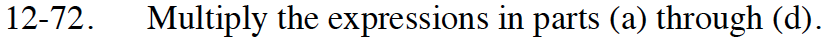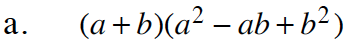Use a generic rectangle to help you. Pay attention to the negative sign.

a³ + b³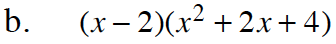Use the same method as part (a).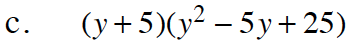Use the same method as part (a).

x³ − 8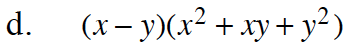Use the same method as part (b).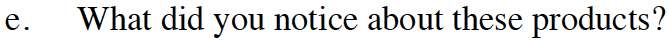Look at your final answers. How many terms are there? And what is the largest exponent?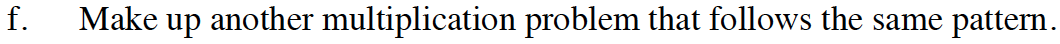The first factor is a binomial. The second factor is a trinomial. Look for a pattern in the terms of the trinomial. Match the sign pattern in one of the problems. Use a generic rectangle to multiply your factors to be sure you have the pattern.